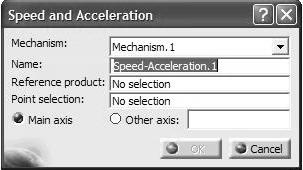# Tutorial : Using Laws in the motion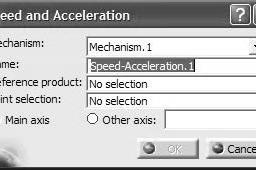1. ### Step 1:

Click on Simulation with Laws icon in the Simulation toolbar .
You will get the following pop up box indication that you need to add at least a relation between a command and the time parameter.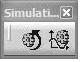2. ### Step 2:

To create the required relation, select the Formula icon from the Knowledge toolbar.

3. ### Step 3:

The pop up box below appears on the screen.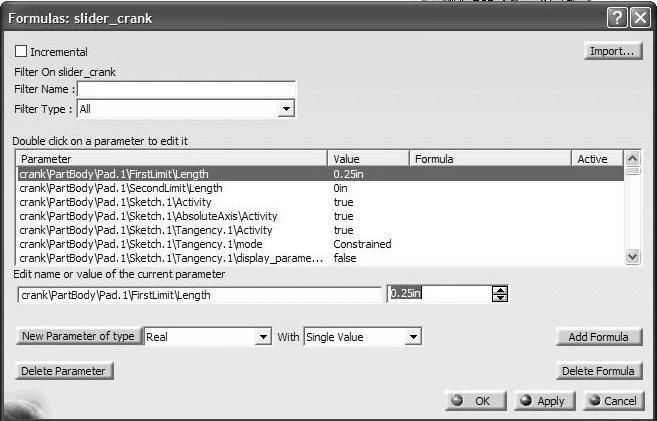4. ### Step 4:

Point the cursor to the Mechanism.1, DOF=0 branch in
the tree and click. The consequence is that only parameters associated with the mechanism are displayed in the Formulas box.
The long list is now reduced to two parameters as
indicated in the box.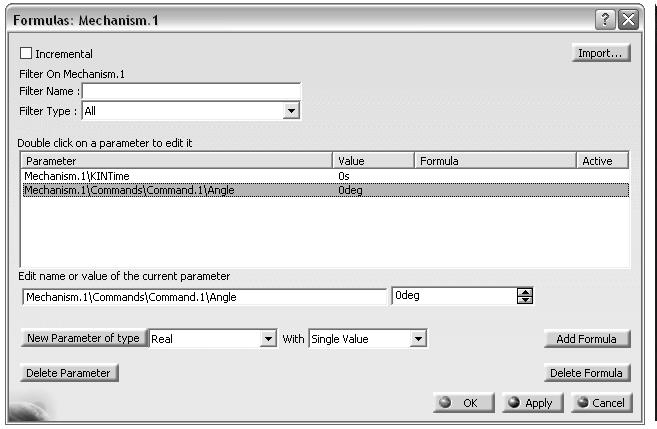5. ### Step 5:

Select the entry Mechanism.1\Commands\Command.1\Angle and press the Add Formula button . This action kicks you to the Formula Editor box.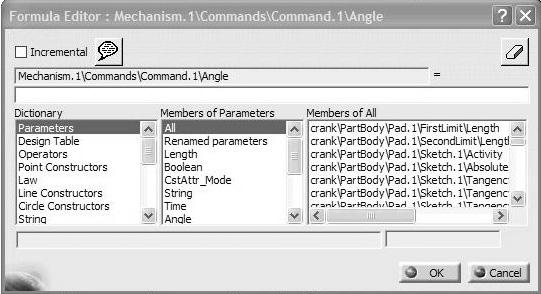6. ### Step 6:

Pick the Time entry from the middle column (i.e. Members of Parameters) then double click on Mechanism.1\KINTime in the Members of Time column.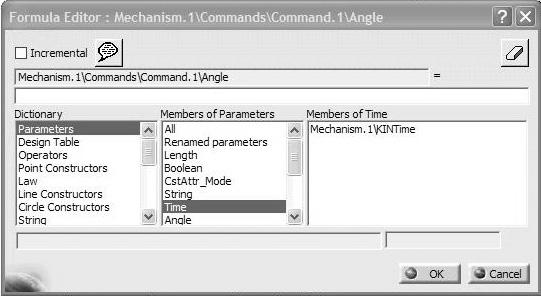7. ### Step 7:

Since angle can be computed as the product of angular velocity (360deg)/(1s) in our case
and time, edit the box containing the right hand side of the equality such that the formula
becomes as shown
The completed Formula Editor box should look as shown below.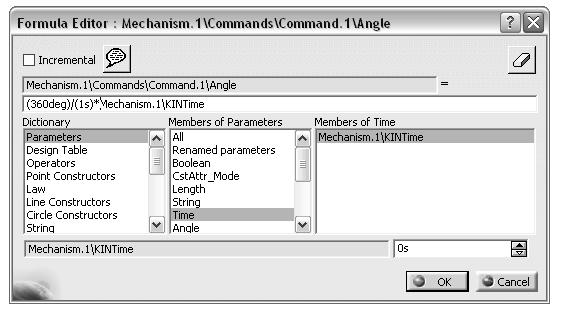8. ### Step 8:

Upon accepting OK, the formula is recorded in the Formulas pop up box as shown below.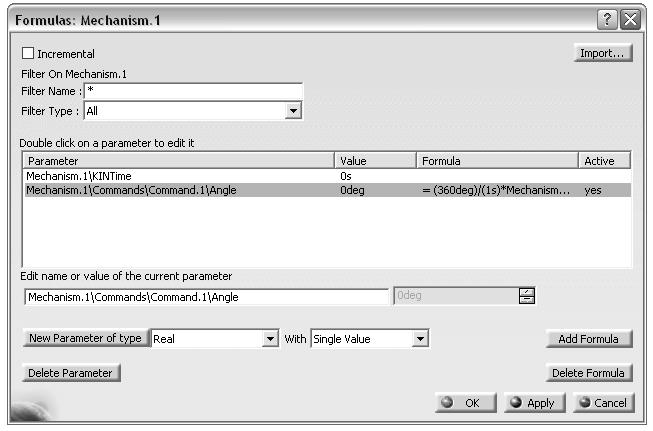9. ### Step 9:

Note that the introduced law has appeared in Law branch of the tree.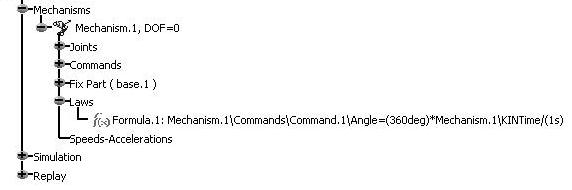10. ### Step 10:

Keep in mind that our interest is to plot the position, velocity and accelerations generated by this motion. To set this up, select the Speed and Acceleration icon from the DMU Kinematics toolbar.
The pop up box below appears on the Screen.